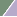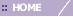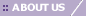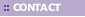# Chebychev

Overview

The Chebychev distance between two points is the maximum distance between the points in any single dimension. The distance between points X=(X1, X2, etc.) and Y=(Y1, Y2, etc.) is computed using the formula:

Maxi |Xi - Yi|

where Xi and Yi are the values of the ith variable at points X and Y, respectively.

The Chebychev distance may be appropriate if the difference between points is reflected more by differences in individual dimensions rather than all the dimensions considered together.

Note that this distance measurement is very sensitive to outlying measurements.

Related Topics:

Clustering Overview

Distance Metrics Overview